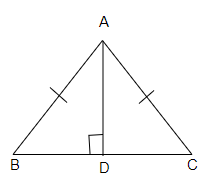# $\mathrm{AD}$ is an altitude of an isosceles triangle $\mathrm{ABC}$ in which $\mathrm{AB}=\mathrm{AC}$. Show that(i) ADbisects BC(ii) $\mathrm{AD}$ bisects $\angle \mathrm{A}$.

Given:

$AD$ is an altitude of an isosceles triangle $ABC$ in which $AB=AC$.

To do:

We have to show that:
(i) $AD$ bisects $BC$
(ii) $AD$ bisects $\angle A$.

Solution:Let us consider $\triangle ABD$ and $ACD$

Given, that $AD$ is the altitude of $\triangle ABD$ and $ACD$

We get, $\angle ADB=\angle ADC=90^o$

We have, $AB=AC$

Since $AD$ is the common side we get,

$AD=DA$

Therefore,

We know that According to the RHS rule if the hypotenuse and one side of a right-angled triangle are equal to the corresponding hypotenuse and one side of another right-angled triangle; then both the right-angled triangle are said to be congruent.

Therefore,

$\triangle ABD \cong \triangle ACD$

We also know that,

From corresponding parts of congruent triangles: If two triangles are congruent, all of their corresponding sides must be equal.

Therefore,

$BD=CD$

Hence, $AD$ bisects $BC$.

(ii) Again from CPCT,

We also know

From corresponding parts of congruent triangles: If two triangles are congruent, all of their corresponding angles must be equal.

Hence,

$AD$ bisects $\angle A$.

Updated on: 10-Oct-2022

28 Views